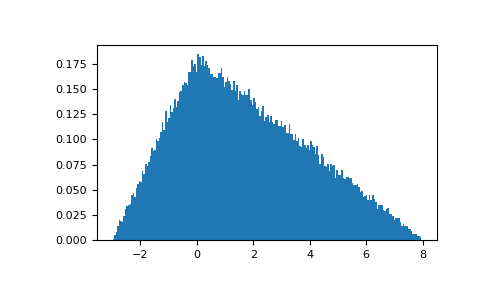# numpy.random.triangular¶

numpy.random.triangular(left, mode, right, size=None)

Draw samples from the triangular distribution over the interval [left, right].

The triangular distribution is a continuous probability distribution with lower limit left, peak at mode, and upper limit right. Unlike the other distributions, these parameters directly define the shape of the pdf.

Note

New code should use the triangular method of a default_rng() instance instead; see random-quick-start.

Parameters
leftfloat or array_like of floats

Lower limit.

modefloat or array_like of floats

The value where the peak of the distribution occurs. The value must fulfill the condition left <= mode <= right.

rightfloat or array_like of floats

Upper limit, must be larger than left.

sizeint or tuple of ints, optional

Output shape. If the given shape is, e.g., (m, n, k), then m * n * k samples are drawn. If size is None (default), a single value is returned if left, mode, and right are all scalars. Otherwise, np.broadcast(left, mode, right).size samples are drawn.

Returns
outndarray or scalar

Drawn samples from the parameterized triangular distribution.

Generator.triangular

which should be used for new code.

Notes

The probability density function for the triangular distribution isThe triangular distribution is often used in ill-defined problems where the underlying distribution is not known, but some knowledge of the limits and mode exists. Often it is used in simulations.

References

1

Wikipedia, “Triangular distribution” https://en.wikipedia.org/wiki/Triangular_distribution

Examples

Draw values from the distribution and plot the histogram:

>>> import matplotlib.pyplot as plt
>>> h = plt.hist(np.random.triangular(-3, 0, 8, 100000), bins=200,
...              density=True)
>>> plt.show()#### Previous topic

numpy.random.standard_t

#### Next topic

numpy.random.uniform